# GMAT Math : DSQ: Calculating the length of an edge of a prism

## Example Questions

### Example Question #1 : Dsq: Calculating The Length Of An Edge Of A Prism

A carpenter is building a box to hold his tools. Find the legth of the second smallest side of the box.

I) The box will have a volume of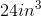.

II) The smallest side is half the length of the longest side and the middle side is three-quarters of the length of the longest side.

Both statements are needed to answer the question.

Statement I is sufficient to answer the question, but statement II is not sufficient to answer the question.

Either statement is sufficient to answer the question.

Statement II is sufficient to answer the question, but statement I is not sufficient to answer the question.

Both statements are needed to answer the question.

Explanation:

Volume of a prism is found by: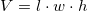We are given the volume in statement I.

We are told how the sides relate in statement II.

Put together these two statements will allow us to set up an equation to find the middle side.

### Example Question #2 : Dsq: Calculating The Length Of An Edge Of A Prism

A pirate wants to hide all of his treasure. He commisions a local woodworker to build him a series of wooden chests of volume of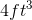. Find the length of the longest side given the following:

I) The shortest side will be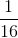the length of the medium side.

II) The middle side will be 2 feet long.

Either statement is sufficient to answer the question.

Statement II is sufficient to answer the question, but statement I is not sufficient to answer the question.

Statement I is sufficient to answer the question, but statement II is not sufficient to answer the question.

Both statements are needed to answer the question.

Both statements are needed to answer the question.

Explanation:

A pirate wants to hide all of his treasure. He commisions a local woodworker to build him a series of wooden chests of volume of. Find the length of the longest side given the following:

I) The shortest side will bethe length of the medium side

II) The middle side will be 2 feet long

Use I) and II) to find the length of the smallest side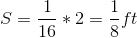Next, use the short and medium sides, along with info in the prompt, to find the last side: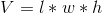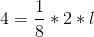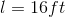### Example Question #3 : Dsq: Calculating The Length Of An Edge Of A Prism

Find the height of a box used to ship a computer, given the following:

I) The computer, along with all the cushioning, will take up a space of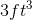.

II) The width of the box will bethe length of the box.

Statement I is sufficient to answer the question, but statement II is not sufficient to answer the question.

Statement II is sufficient to answer the question, but statement I is not sufficient to answer the question.

Either statement is sufficient to answer the question.

Both statements are needed to answer the question.

Explanation:

Find the height of a box used to ship a computer, given the following:

I) The computer, along with all the cushioning, will take up a space ofII) The width of the box will bethe length of the box

Begin by recalling the volume of a rectangular prism formula: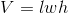Where l,w and h are length, width and height.

Next, use II) to set up a relationship between w and l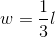Then, use the voume formula: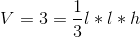As you can see, we still have two unknowns, and no way of finding either.

Therefore, we do not have information.

Tired of practice problems?

Try live online GMAT prep today.